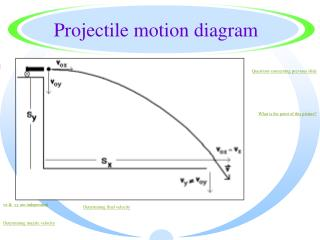DownloadDownload PresentationProjectile motion diagram

# Projectile motion diagram

Download Presentation## Projectile motion diagram

- - - - - - - - - - - - - - - - - - - - - - - - - - - E N D - - - - - - - - - - - - - - - - - - - - - - - - - - -
##### Presentation Transcript

1. Projectile motion diagram Questions concerning previous slide What is the point of this picture? vx & vy are independent Determining final velocity Determining muzzle velocity

2. Questions concerning previous slide • What does each of the following designate? vox, vx, voy, vy, dx, dy, v? Say it in words. • Why does vx = vox? • What is the value of voy? • What is the value of vy? • What is the value of vox & vx? • What is the value of v? Diagram

3. vox • vox = the initial velocity in the horizontal direction. • vox = muzzle velocity of, in this case, the cannon

4. Vx • vx is the final velocity in the horizontal direction or the velocity @ any point in time in the horizontal direction.

5. voy • Voy is the initial velocity of the projectile in the vertical direction.

6. vy • vy is the final velocity in the vertical direction or the velocity in the vertical direction @ any point in time.

7. dx dx is the distance traveled by the projectile in the horizontal direction. Also called “range” & sometimes designated “R”.

8. dy • dy is the heighth from which the projectile is fired or the vertical distance the projectile falls.

9. v • v = the final velocity of the projectile just before it strikes the ground or the velocity of the projectile @ any point in time. That is, polar form of the vector w/ vx & vy being the rectangular form.

10. vx = vox • vx = vox b/c there is no acceleration in the horizontal direction if we neglect air resistance (which is negligible with a cannonball or for any projectile over short vertical falls).

11. Value of voy • voy = 0 since the cannonball was projected horizontally.

12. Values Value of vy Value of vox & vx vx = vox = dx/tx • vy = voy2 + 2gdy • Do you understand how we arrived @ this?

13. Value of v • v = vx + vy • i.e. the vector sum • vx & vy = rectangular form • v = polar form

14. What is the point of this picture? • Also se fig. 6-1 p. 148 of your text, essentially the same picture. • This picture depicts a device that shoots & drops 2 balls simultaneously. • Projectile motion diagram

15. vx & vy are independent • The velocity in the vertical direction is unaffected by what is happening in the horizontal direction. Return to the previous slide & notice that the vertical posn. of the projected ball & dropped ball is always the same! • Projectile motion diagram Last slide

16. vx & vy • Velocity in the horizontal direction is constant. • Velocity in the vertical direction is inconstant, vy ≠ voy. Velocity in the vertical direction varies in accordance w/ g & time.

17. Determining muzzle velocity Muzzle velocity is the velocity w/ which a bullet emerges from the barrel of a gun. • vox = vx • & vx = dx/tx • Therefore, we must know dx & tx • dx can be measured. Projectile motion diagram

18. Determining muzzle velocity - cont. • & tx = ty How fast something falls is independent of vo. This is true as long as vo is totally in the x direction i.e. voy = 0. Projectile motion diagram

19. It may appear to be affected less when moving @ faster speeds but this is only b/c of the vx/vy ratio • Since tx = ty • ty = ? • dy = voyt + 1/2 gty2 • ty = 2dy/g • Not we can calculate vx. vx = dx/tx Projectile motion diagram

20. Determining final velocity • v = vx + vy • vx - calculated on previous slides • vy can be calculated (instant before it hits the ground). • vy2 = voy2 + 2gdy • vy = 2gdy • Projectile motion diagram

21. Determining final velocity - cont. • tan Q = vy/vx • Magnitude: • cos Q = vx/v or sin Q = vy/v • v = vx/cos Q or v = vy/sin Q • Better yet since we know vx & vy Simply R->P • Projectile motion diagram

22. Air resistance • So far air resistance has not been considered. • There are 2 ways air resistance affects projectile motion. • Decrease vx • Decrease vy • Think about it!

23. Ballisitics • The study of projectile motion which considers all forces working on a projectile -- that is, it considers air resistance as well as gravity.

24. Key concept • The velocity in the vertical direction is unaffected by what is happening in the horizontal direction.